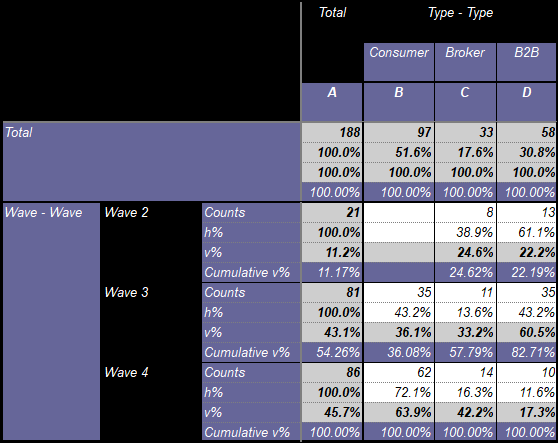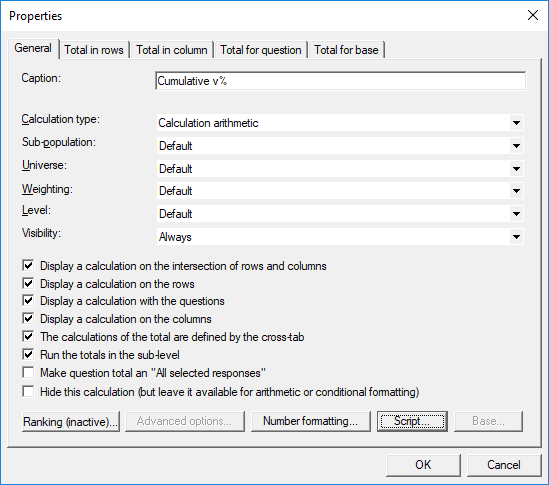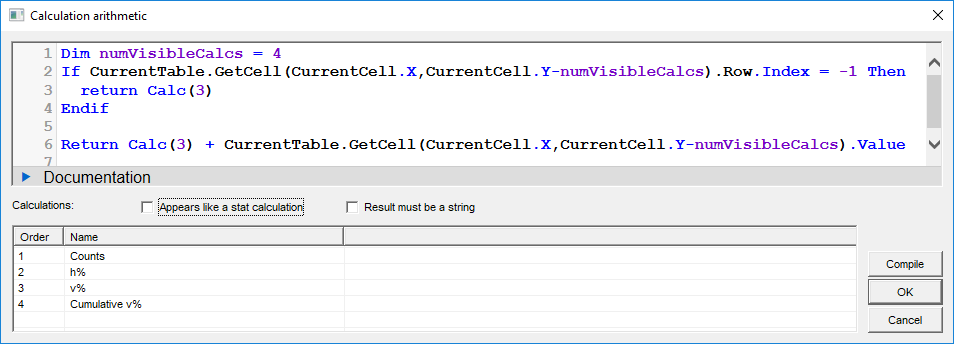Scroll

# Summing Calculations (Cumulative %)

Follow
 Summary This article will demonstrate more of the scripts that can be used in calculation arithmetic. Here we will concentrate on how to sum up calculations, specifically, to give a cumulative vertical % calculation Applies to Askia Analyse. Written for Researchers, Developers, Data Processors. Keywords Cumulative, Crossed calculation, Table arithmetic, Forloops, Coordinates, data, Calculation arithmetic, Percentage down, vertical %.

The .qes file and portfolio containing the examples discussed is attached: Table Arithmetic Examples 7.zip.

In Analyse, there is a pre-set calculation for Cumulative % on flat calculations i.e. table with only variable in rows (no columns). However, if we want to do this with columns involved (crossed calculations) then there is no pre-set option.

We can get around this by using table arithmetic to add a calculation which will sum up each pre-set vertical %.I will now talk through the steps / syntax used to achieve the above table where we sum v% into a new calculation we have added, 'Cumulative %'.

First insert a calculation of type: Calculation arithmeticHit the script button and enter the syntax shown below (and contained in the example file).The points of note here are as follows:

We want to identify when we are in the first response row and use the inbuilt vertical % calculation for this. i.e. because for the first response % = cumulative %.

Then for each subsequent row, we add the v% to what we had before (in the previous cumulative % cell)

Can we use If CurrentCell.Row.Index1 Then to identify when we are in the first response row?

Row.Index is a sequential number assigned to each response in each variable in the rows. The 1st response of the 1st variable in the rows will have index = 1. The 2nd response of the 1st variable in the rows will have index = 2 etc. On any total row this index is = -1.

We can't really use this syntax to identify the first response. In the above table we tabulate the wave variable. The first response of this variable is Wave 1 (Row.Index = 1). However, the first visible response is Wave 2 (Row.Index = 2). So we need to account for the first response not always being selected.

Furthermore, if I have more than one variable in my row (and they are split one tab per question in rows) then on the second tab, again, my row indexes do not begin from 1.

But we can use something else:

If CurrentTable.GetCell(CurrentCell.X,CurrentCell.Y-numVisibleCalcs).Row.Index = -1 Then

For the first row, we know the current cell (CurrentCell.X,CurrentCell.Y) can have a variable row index. But if we go to the row above the first row (i.e. a total row) we know that it will always have a row index of -1. So we do that by amending the y-coordinate (CurrentCell.X,CurrentCell.Y-numVisibleCalcs) and checking the row index at that location.

So when the condition in the if statement is true, we know we are on the first row and we can return the inbuilt v% [Calc(3)] as the first value.

Return Calc(3)
End if

Then for each subsequent row response we return the inbuilt v% for that cell + the cumulative % total up to that row response.

There is a detailed article about Row, Column & Edge Index keywords here.